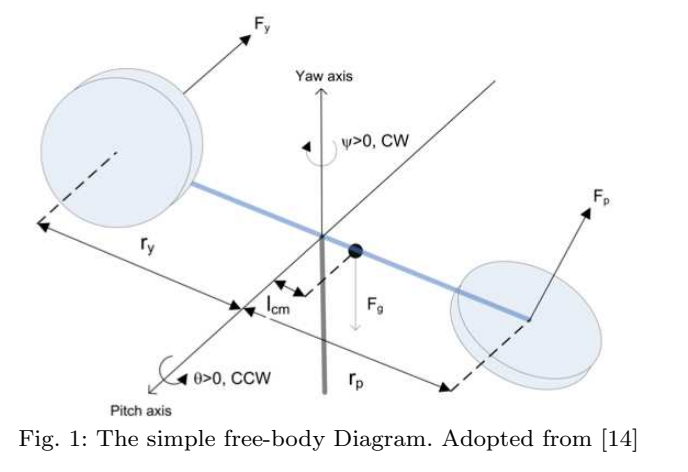Conference Paper

# PID Controller for 2-DOFs Twin Rotor MIMO System Tuned with Particle Swarm Optimization

By
Azar A.T.
Sayed A.S.
Shahin A.S.
Elkholy H.A.
Ammar H.H.

This paper presents the modelling and control of a 2-DOFs Twin rotor multi input multi output (MIMO) system which is a laboratory setup resembling the dynamics of a helicopter. In this paper, the system modelling process is done using the common conventional mathematical model based on Euler-Lagrange method. The transfer functions of the model are used in the different tuning methods to reach the optimal PID gain values. The study uses conventional Proportional-Integral (PI) and Proportional-Integral-Derivative (PID) controllers to obtain a robust controller for the system. Particle Swarm optimization (PSO) technique was used to tune the controller parameters. A state space model is obtained considering some design assumptions and simplifications. Statistical measurement and convergence analysis is evaluated for the optimization of gain parameters of the PID controller for 2-DOF Twin rotor system using PSO by iteratively minimizing integral of squared error (ISE) and integral of time multiplied by the squared error (ITSE). The results are verified through simulations and experiments. © Springer Nature Switzerland AG 2020.